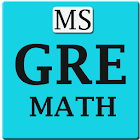Master GRE Math

All Android applications categories

All Android games categories# Master GRE Math

0 0

## Screenshots

Description

Based on the popularity of our app Master Algebra which is among Top 5 free apps for algebra in Google play android market, We have released Master GRE Math , Master GMAT Math & Master SAT Math.

Are you applying for grad school? Need help mastering the GRE? Try out this unconventional method on go. Think out of the box & beat the GRE in first attempt. Brush up your math skills.

Master GRE Math helps you excel in the math section of GRE(Graduate Record Examination) test. This app has Tutorial, Practice Exercise, Practice Test & GRE math challenge.

Covers following topics.

Arithmetic
• Order of Operation
• Least Common Multiples(LCM)
• Greatest Common Factors(GCF)
• Fraction
• Decimal
• Percentage
Algebra
• Types of Number like real number, integer, negative number, complex number
• Manipulation of Real Number, Negative Number, Complex Number
• Properties of Number
• Ratio & Proportion
• Integer exponents/real /rational exponents
• Rational exponent
• Monomial/binomial/polynomial
• Manipulation of polynomials
• Factoring polynomial
• Variable, Expression & Equation
• Equation with radical, Absolute value of equation
• Rational Expression;
• Manipulation of rational expression
• Inequality
• Linear, Polynomial &Rational Inequalities
• Absolute value of Inequality
Geometry
• Lines, angles & triangle
• Types & properties of triangle
• Area & perimeter of circle
• Solids & co-ordinate geometry
• Area & perimeter of rectangle, square, rhombus, parallelogram.

Probability & Statistics
• Mean median, mode & data analysis

Practice exercise has 100 questions with step by step solution and explanation.25 questions in each section.

Practice Test has 100 different questions having timer which will give you a feel of real test.

GRE Math Challenge has also 100 questions. Take the challenge after you finish tutorial, practice exercise & practice test. This is basically for comparing your performance with others.

Master GRE Math app will display 50 excellent performers in Global Champion section. You can come to know who else took the challenge and how they performed in compare section even which country they are from.

Reports get stored in our database. You or your parent can check anytime and it can be sent via email too.

How this app is different than others?
Answer: It not only helps you practice but also you can come to know how good you are in comparison to others. It displays the report for all who took up the challenge.

Disclaimer: GRE is a registered trademark of Educational Testing Services (ETS). ETS neither sponsors nor endorses this product or any of its content.